• 最近接触了一点雅克比的东西，以前学习雅克比矩阵和雅克比行列式是在高数上，就知道个二重积分的时候可以用一下，其他的真没遇到过。最近在学习随机过程，在涉及到随机变量转化求解概率密度函数时，猛然冒出雅克比...

最近接触了一点雅克比的东西，以前学习雅克比矩阵和雅克比行列式是在高数上，就知道个二重积分的时候可以用一下，其他的真没遇到过。最近在学习随机过程，在涉及到随机变量转化求解概率密度函数时，猛然冒出雅克比行列式让我刮目相看，于是再次学习这些东西。

首先介绍定义，雅克比矩阵是一阶偏导数以一定的方式排列成的矩阵，当其实方阵时，行列式称为雅克比行列式。设有m个n元函数组成的函数组：，称之为函数组。我们对这个函数组取一阶导数，获得下面的雅克比矩阵：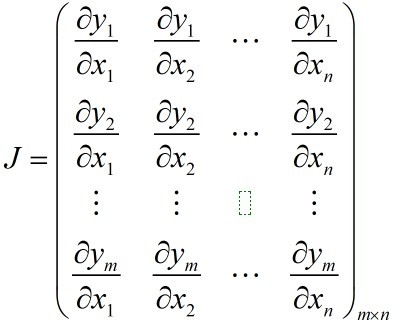如果m=n，那么J就是一个方阵，于是我们就得到对应的雅克比行列式：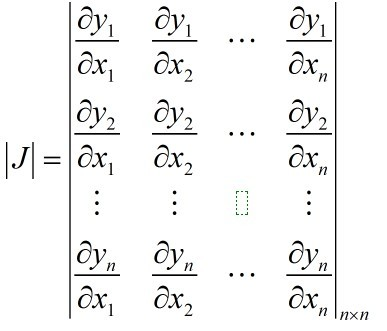首先讨论雅克比矩阵，凡是矩阵都可以看做是一个线性空间之间的转换工具，这里也不例外，我们将雅克比矩阵看做是将点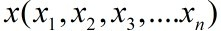转化到点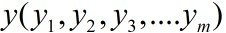，或者说是从一个n维的欧式空间转换到m维的欧氏空间。这里需要强调的是不要和hessian
阵混合，后者也是梯度矩阵，针对的是多元函数的二阶偏导数构成的方块阵。

下面介绍雅克比矩阵和雅克比行列式的数学和物理意义。

Eg1.雅克比矩阵可以用来体现一个可微方程与给定的某个点的最佳线性逼近，也可以理解为某点的一阶展开，因为雅克比矩阵类似多元函数的导数，只是这里的函数是函数组。雅克比矩阵的第i行的转置就是函数yi的梯度。例如在某点p处可微，那么我们将有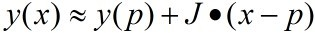。

Eg2.坐标变换

球坐标与直角坐标的变换公式如下：

实现了将球空间转化为笛卡尔空间。我们得到的雅克比矩阵是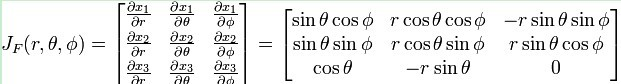更加具体的参考blog：

http://blog.sina.com.cn/s/blog_4062094e0100c2p1.html

这个需要强调的是在这个例子中雅克比矩阵更加准确的体现的是其微分形式，反应了原始空间微小变化引发的值域空间的变化的敏感度。

Eg3. 雅克比行列式的性质。雅克比行列式可以看做是空间的坐标变换时对应的面积（或者体积）元素的伸缩系数

在应用到多重积分的变量替换是最常用到的。例如对于二重积分：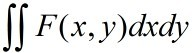---------1，

我们进行变量替换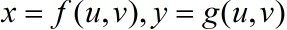-------2，于是将公式2代入到1中我们得到：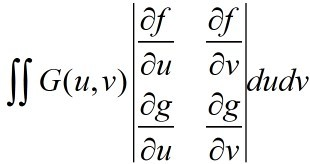，在这里。我们做这么麻烦的转化只是为了将来的运算方便，一种情况是在x，y不好运算，比如我们用极坐标运算来代替直角坐标运算。第二种是，x，y的运算未知，而我们已   经知道了u，v的运算以及两者之间转换关系。

总之，雅克比行列式表示不同坐标下的转换尺度。

转自http://blog.csdn.net/carrierlxksuper/article/details/12453307

展开全文• 矩阵求导是机器学习中常见的运算方法，研究对象包括标量，向量和矩阵，求导分为标量向量、矩阵求导，向量标量、向量、矩阵求导，矩阵对标量、向量、矩阵求导。 根据个人理解和经验，机器学习中的优化目标一般是...
Jacobian矩阵 梯度矩阵 矩阵偏导与微分 常见公式
矩阵求导是机器学习中常见的运算方法，研究对象包括标量矩阵，求导分为标量矩阵求导，矩阵求导。
根据个人理解和经验，机器学习中的优化目标一般是一个由向量或矩阵运算得到的标量，因此应该重点关注标量对向量和矩阵的求导。
本文总结了矩阵求导的定义和常见公式，主要内容来自张贤达《矩阵分析与应用（第二版）》的第三章。
Jacobian矩阵
矩阵导数可以理解成实值标量函数、实值向量函数、实值矩阵函数对于向量或矩阵中的每一个元素的偏导，是由一系列偏导组成的。
若有$m$维列向量$x\in \mathbb{R}^{m\times 1}$，变元为$x$的实值标量函数$f(x)$在$x$处的偏导向量定义为：
$\frac{\partial f(x)}{\partial x^T} =[\frac{\partial f}{\partial x_1},\frac{\partial f}{\partial x_2},\cdots ,\frac{\partial f}{\partial x_m}]$
若有矩阵$X\in \mathbb{R}^{m\times n}$，变元为$X$的实值标量函数$f(X)$在$X$处的Jacobian矩阵定义为：
$\frac{\partial f(X)}{\partial x^T} =\left[ \begin{matrix} \frac{\partial f(X)}{\partial X_{11}} & \cdots & \frac{\partial f(X)}{\partial X_{m1}} \\ \vdots & \ddots & \vdots \\ \frac{\partial f(X)}{\partial X_{1n}} & \cdots & \frac{\partial f(X)}{\partial X_{mn}} \\ \end{matrix} \right]\in \mathbb{R}^{n\times m}$
对于矩阵$X\in \mathbb{R}^{m\times n}$，实值矩阵函数$f(X)\in \mathbb{R}^{p\times q}$在$X$处的Jacobian矩阵定义为：
$\frac{\partial f(X)}{\partial X^T} =\left[ \begin{matrix} \frac{\partial f(X)_{11}}{\partial X_{11}} & \cdots & \frac{\partial f(X)_{11}}{\partial X_{m1}} & \cdots & \frac{\partial f(X)_{11}}{\partial X_{mn}} \\ \vdots & \ddots & \vdots &\ddots & \vdots\\ \frac{\partial f(X)_{p1}}{\partial X_{11}} & \cdots & \frac{\partial f(X)_{p1}}{\partial X_{m1}} & \cdots & \frac{\partial f(X)_{p1}}{\partial X_{mn}} \\ \vdots & \ddots & \vdots &\ddots & \vdots\\ \frac{\partial f(X)_{pq}}{\partial X_{11}} & \cdots & \frac{\partial f(X)_{pq}}{\partial X_{m1}} &\cdots & \frac{\partial f(X)_{pq}}{\partial X_{mn}} \\ \end{matrix} \right]\in \mathbb{R}^{pq\times mn}$
这个Jacobian矩阵是分别对$f(X)$和$X$做向量化然后逐元素求偏导得到的。这里的$f(X)$和$X$都是按列展开的。
有了这个通用公式，其他关于向量的各种Jacobian矩阵也都有定义了。
梯度矩阵
实值标量函数$f(x)$在列向量变元$x\in \mathbb{R}^{m\times 1}$处的梯度向量定义为：
$\frac{\partial f(x)}{\partial x} =[\frac{\partial f(x)}{\partial x_1},\cdots,\frac{\partial f(x)}{\partial x_m}]^T$
注意这是个列向量。
实值标量函数$f(X)$在矩阵变元$X\in \mathbb{R}^{m\times n}$处的梯度矩阵定义为：
$\frac{\partial f(X)}{\partial X} =\left[ \begin{matrix} \frac{\partial f(X)}{\partial X_{11}} & \cdots & \frac{\partial f(X)}{\partial X_{1n}} \\ \vdots & \ddots & \vdots \\ \frac{\partial f(X)}{\partial X_{m1}} & \cdots & \frac{\partial f(X)}{\partial X_{mn}} \\ \end{matrix} \right]\in \mathbb{R}^{m\times n}$
实值矩阵函数$f(X)\in \mathbb{R}^{p\times q}$在矩阵变元$X\in \mathbb{R}^{m\times n}$处的梯度矩阵定义为：
$\frac{\partial f(X)}{\partial X} =\left[ \begin{matrix} \frac{\partial f(X)_{11}}{\partial X_{11}} & \cdots & \frac{\partial f(X)_{p1}}{\partial X_{11}} & \cdots & \frac{\partial f(X)_{pq}}{\partial X_{11}} \\ \vdots & \ddots & \vdots &\ddots & \vdots\\ \frac{\partial f(X)_{11}}{\partial X_{m1}} & \cdots & \frac{\partial f(X)_{p1}}{\partial X_{m1}} & \cdots & \frac{\partial f(X)_{pq}}{\partial X_{m1}} \\ \vdots & \ddots & \vdots &\ddots & \vdots\\ \frac{\partial f(X)_{11}}{\partial X_{mn}} & \cdots & \frac{\partial f(X)_{p1}}{\partial X_{mn}} &\cdots & \frac{\partial f(X)_{pq}}{\partial X_{mn}} \\ \end{matrix} \right]\in \mathbb{R}^{mn\times pq}$
相同函数与变元对应的Jacobian矩阵和梯度矩阵互为转置关系。
不知道是张老的书写的不够简明扼要，还是我没认真看，这么简单的定义我看了好久才搞明白。书中指出，在流行计算、几何物理、微分几何等领域，行向量偏导向量和Jacobian矩阵是最自然的选择，在最优化和许多工程问题中，梯度向量和梯度矩阵是最自然的选择。这也符合我的一些经验，梯度矩阵看起来要比Jacobian矩阵顺眼很多。
一般见到的矩阵导数是梯度矩阵的形式。 说白了Jacobian矩阵是对$X^T$求导得到的，梯度矩阵是对$X$求导得到的。
矩阵偏导和梯度计算法则
一般说的矩阵导数就是梯度矩阵或向量。根据定义可有如下常用运算法则：

若$c$为常数，$\frac{\partial c}{\partial X}=O_{m\times n}$，$O_{m\times n}$是$m$行$n$列的0矩阵.
$\frac{\partial [c_1f(X)+c_2g(X)]}{\partial X}=c_1 \frac{\partial f(X)}{\partial X}+c_2 \frac{\partial g(X)}{\partial X}$
$\frac{\partial [f(X)g(x)]}{\partial X}=g(X)\frac{\partial f(X)}{\partial X}+f(X)\frac{\partial g(X)}{\partial X}$
$\frac{\partial [f(X)g(X)h(X)]}{\partial X}=g(X)h(X)\frac{\partial f(X)}{\partial X}+f(X)h(X)\frac{\partial g(X)}{\partial X}+f(X)g(X)\frac{\partial h(X)}{\partial X}$
$\frac{\partial [f(X)/g(X)]}{\partial X}=\frac{1}{g^2(X)}[g(X)\frac{\partial f(X)}{\partial X}-f(X)\frac{\partial g(X)}{\partial X}]$
$\frac{\partial g(f(X))}{\partial X}=\frac{dg(f(X))}{df(X)}\frac{\partial f(X)}{\partial X}$
求导链式法则：$\frac{\partial g(f(X))}{\partial X}=\frac{dg(y)}{dy} \frac{\partial f(X)}{\partial X}$

此外在计算以向量和矩阵为变元的函数的偏导时，有个重要的独立性基本假设，即向量和矩阵中的各个元素是相互独立的，用公式表示为：
$\frac{\partial x_i}{\partial x_j}=\left\{ \begin{array}{l} 1,if\ i=j \\ 0,else\end{array}\right.$
以及：
$\frac{\partial x_{kl}}{\partial x_{ij}}=\left\{ \begin{array}{l} 1,if\ k=i\ and\ l=j \\ 0,else\end{array}\right.$
举个根据定义求解梯度矩阵的例子，求实值函数$f(X)=a^TXX^Tb$在矩阵变元$X$处的梯度矩阵，$a,b$均为$n$维列向量：
$a^TXX^Tb=\sum_{k=1}^m\sum_{l=1}^na_k(\sum_{p=1}^nx_{kp}x_{lp})b_l$
然后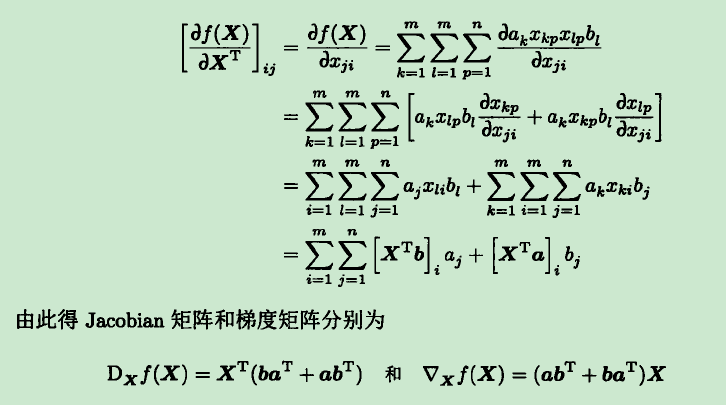根据定义就是这样求解的。
矩阵微分以及与一阶导数的关系：Jacobian矩阵的辨识
矩阵微分的定义为：
$dX=[dX_{ij}]_{i,j=1}^{m,n}$
标量对标量的导数是用微分定义的，标量$f$对标量$x$的导数$f'(x)$满足$df=f'(x)dx$。而实值标量函数$f(x)$对向量$x$的导数与微分的关系，可以表示为（此表示的证明书上有）：
$df(x)=\sum_{i=1}^n\frac{\partial f(x)}{\partial x_i}dx_i=\frac{\partial f(x)}{\partial x}^Tdx$
即$f(x)$的微分与$x$中每个元素的微分都有关，$\frac{\partial f(x)}{\partial x}$即为标量$f$对向量$x$的梯度向量，是一个向量。
同样，实标量函数$f(X)$对矩阵$X\in\mathbb{R}^{m\times n}$求导时，$f(X)$的微分也与$X$中每个元素有关，表示为（此表示的证明书上有）：
$df(X)=\sum_{i=1}^m\sum_{j=1}^n\frac{\partial f(X)}{\partial X_{ij}}dX_{ij}=tr(\frac{\partial f(X)}{\partial X}^TdX)$
其中$tr$表示的是矩阵求迹运算，$\frac{\partial f(X)}{\partial X}$表示$f(X)$对$X$的梯度矩阵。后一个等号成立的原因是矩阵迹运算有如下性质：
$tr(A^TB)=\sum_{i,j}A_{ij}B_{ij}$
即$A^TB$的迹等于$A$与$B$中对应元素乘积的和。
这部分给出了微分矩阵与实标量函数对向量和矩阵变元的Jacobian矩阵（向量）和梯度矩阵（向量）的关系，这种关系也可以用来求实标量函数对向量和矩阵变元的Jacobian矩阵和梯度矩阵，这种关系称为Jacobian矩阵的辨识。
书上还给了实矩阵函数对矩阵变元导数与微分矩阵的辨识关系，以及二阶导数与微分矩阵的关系（Hessian矩阵的辨识，Hessian矩阵即矩阵二阶导），不过由于我不是很关注，所以没写在这里。
矩阵微分运算法则
这里给出一些求矩阵微分和迹的运算法则：

$d(X+Y)=dX+dY,d(XY)=(dX)Y+X(dY)$
$d(X^T)=(dX)^T$
$dA=0$，$A$为常数矩阵.
$d(aX)=ad(X)$，$a$为常数.
$d(AXB)=A(dX)B$，$A,B$为常数矩阵.
$d(f(X)g(X)h(X))=(df(X))g(X)h(X)+f(X)(dg(X))h(X)+f(X)g(X)(dh(X))$
$dtr(X)=tr(dX)$
$d|X|=|X|tr(X^{-1}dX)$，行列式的微分

举个用微分与梯度矩阵的关系求梯度矩阵的例子，求$f(X)=tr(XAXB)$对于矩阵$X$的梯度矩阵：
$dtr(XAXB)=tr(d(XAXB))\\ =tr[(dX)AXB+XA(dX)B]\\ =tr[(AXB+BXA)dX]$
因此得梯度矩阵：
$\frac{\partial tr(XAXB)}{\partial X}=(AXB+BXA)^T$
常用矩阵求导公式总结
在Matrix Cookbook第二章Derivatives里面有很多，这里取常见的一些总结如下。我们用$\frac{\partial f(X)}{\partial X}$表示$f(X)$在$X$处的导数（即梯度矩阵），大写字母为矩阵，小写字母为列向量，则有：

$\frac{\partial a^TXb}{\partial X}=ab^T$
$\frac{\partial a^TX^Tb}{\partial X}=ba^T$
$\frac{\partial x^TAx}{\partial x}=(A+A^T)x$
$\frac{\partial a^TXX^Tb}{\partial X}=(ab^T+ba^T)X$
$\frac{\partial a^TX^TXb}{\partial X}=X(ab^T+ba^T)$
$\frac{\partial b^TX^TDXc}{\partial X}=DXbc^T+DXcb^T$
$\frac{\partial (Xb+c)^TD(Xb+c)}{\partial X}=(D+D^T)(Xb+c)b^T$
$\frac{\partial (Ax+b)^TC(Dx+e)}{\partial x}=D^TC^T(Ax+b)+A^TC(Dx+e)$

迹的导数：

$\frac{\partial tr(X)}{\partial X}=I$
$\frac{\partial tr(XA)}{\partial X}=A^T$
$\frac{\partial tr(X^TA)}{\partial X}=A$
$\frac{\partial tr(AX^T)}{\partial X}=A$
$\frac{\partial tr(AXB)}{\partial X}=A^TB^T$
$\frac{\partial tr(AX^TB)}{\partial X}=BA$
$\frac{\partial tr(BX^TX)}{\partial X}=XB^T+XB$
$\frac{\partial tr(AXBX)}{\partial X}=A^TX^TB^T+B^TX^TA^T$
$\frac{\partial tr(B^TX^TCXB)}{\partial X}=C^TXBB^T+CXBB^T$
$\frac{\partial tr(AXBX^TC)}{\partial X}=A^TC^TXB^T+CAXB$
$\frac{\partial tr(AX^TBXC)}{\partial X}=BXCA+B^TXA^TC^T$
$\frac{\partial tr[(AXB+C)(AXB+C)^T]}{\partial X}=2A^T(AXB+C)B^T$

范数的导数：

$\frac{\partial \|x\|_2^2}{\partial x}=\frac{\partial x^Tx}{\partial x}=2x$
$\frac{\partial }{\partial x}\|x-a\|_2=\frac{x-a}{\|x-a\|_2}$
$\frac{\partial \|X\|_F^2}{\partial X}=\frac{\partial }{\partial X}tr(XX^H)=2X$

行列式的导数：

$\frac{\partial det(X)}{\partial X}=det(X)(X^{-1})^T$
$\frac{\partial det(AXB)}{\partial X}=det(AXB)(X^{-1})^T=det(AXB)(X^{T})^{-1}$

暂时就这些，以后还有别的再补充。Matrix Cookbook上还有许多其他公式，也有的公式没有给出。如前所述，目前关注的还是实标量函数对矩阵和向量的导数，许多实矩阵函数对矩阵的导数的公式这里没有给出。


展开全文• n阶矩阵（方阵）AAA的行列式是一个标量，如何计算就不啰嗦了. 1、物理意义 An×nA^{n\times n}An×n表示一个n维空间到n维空间的线性变换: f:Rn→Rnf:R^n\to R^nf:Rn→Rn, 是一个压缩或拉伸， 即scale操作。而{det...
note行列式：$det(A)$奇异矩阵矩阵范数条件数AdaGrad
行列式：$det(A)$
n阶矩阵（方阵）$A$的行列式是一个标量，如何计算就不啰嗦了.
1、物理意义
$A^{n\times n}$表示一个n维空间到n维空间的线性变换: $f:R^n\to R^n$, 是一个压缩或拉伸， 即scale操作。而$det(A)$就是这个缩放尺寸。
（1） 假想原来空间中有一个$n$维的立方体（随便什么形状），其中立方体内的每一个点都经过这个线性变换，变成$n$维空间中的一个新立方体。
（2） 原来立方体有一个体积$V_{1}$，新的立方体也有一个体积$V_{2}$ 。
（3） 行列式$det(A)$是一个数对不对？这个数其实就是 $V_{2} \div V_{1}$ 。
2、性质
$det(A)\times det(B)=det(AB)$
奇异矩阵
奇异矩阵就是说该矩阵不是满秩的。

首先，看这个矩阵是不是方阵。
再看此矩阵的行列式|A|是否等于0，若等于0，称矩阵A为奇异矩阵；  若不等于0，称矩阵A为非奇异矩阵。
可逆矩阵和非奇异矩阵是一个意思。
A矩阵不可逆（奇异矩阵）的充要条件：
<=> |A| = 0
<=> A的列(行)向量组线性相关
<=> R(A)<n
<=> AX=0 有非零解
<=> A有特征值0.
<=> A不能表示成初等矩阵的乘积
<=> A的等价标准形不是单位矩阵

矩阵范数
1-范数：$||A||_1 = \max_j\sum_{i=1}^m|a_{i,j}|$, 列和范数，即所有矩阵列向量绝对值之和的最大值，matlab调用函数norm(A, 1)， python调用为np.linalg.norm(A, ord=1) 。
2-范数：$||A||_2 = \sqrt{\lambda_1}，\lambda_1$为$A^TA$的最大特征值，又称为谱范数matlab调用函数norm(x, 2)， python调用为np.linalg.norm(A, ord=2) 。
$\infty$-范数：$||A||_\infty = \max_i\sum_{j=1}^N|a_{i,j}|$，行和范数，即所有矩阵行向量绝对值之和的最大值，matlab调用函数norm(A, inf), python调用为np.linalg.norm(A, ord=np.inf) 。
F-范数：$||A||_F=\left(\sum_{i=1}^m\sum_{j=1}^n|a_{i,j}|^2\right)^{\frac{1}{2}}$，Frobenius范数，即矩阵元素绝对值的平方和再开平方，matlab调用函数norm(A, ’fro‘)，python调用为np.linalg.norm(A, ord=‘fro’) 。
核范数：$||A||_* = \sum_{i=1}^{n}\lambda_i, \quad \lambda_i$是A的奇异值，即奇异值之和。
条件数
条件数定义为：$cond(A)=||A||*||A^{-1}||$，$||A||$是矩阵的某一种范数。
Condition number是一个矩阵（或者它所描述的线性系统）的稳定性或者敏感度的度量，如果一个矩阵的condition number在1附近，那么它就是well-conditioned的，如果远大于1，那么它就是ill-conditioned的，如果一个系统是ill-conditioned的，它的输出结果就不要太相信了。
这是因为若$y=Ax$，给$x$一个微小的扰动量$\Delta x$，输出的结果会变化很大，即$y'=A(x+\Delta x)$，$|y'-y|$是一个难以容忍的值。
'''
优点：抑制梯度大的维度的下降速度，增大梯度小的维度的下降速度
缺点：随着迭代，步长越来越小，在非凸问题上容易卡在鞍点和局部极小值
'''
while True:
x -= learning_rate * dx / (np.sqrt(grad_squared) + 1e-7)

对于条件数很大的矩阵，比如一个维度有很大的梯度，另一个维度有很小的梯度，我们除以这个梯度平方的累加和会使梯度小的维度学习率增大，而梯度大的维度学习率降低。
【参考链接】
1、奇异值和特征值
2、计算矩阵/向量的范数：numpy.linalg.norm()
3、cs231n优化算法


展开全文优化算法 线性代数
• 一维f(x)，一阶导数构成的向量为梯度向量g(x)； 多维时，一阶导数构成的矩阵为Jacobian矩阵； 雅可比矩阵梯度差别在于：雅可比矩阵是一阶导数的矩阵梯度是一阶导数构成的方向向量。 ...
一维f(x)，一阶导数构成的向量为梯度向量g(x)；
多维时，一阶导数构成的矩阵为Jacobian矩阵；
雅可比矩阵与梯度差别在于：雅可比矩阵是一阶导数的矩阵，梯度是一阶导数构成的方向向量。


展开全文• 若向量值函数$$m=n$$，则其Jacobi矩阵行列式为Jacobi行列式，记作$$\dfrac{D(f_1,f_2,\cdots,f_m)}{D(x_1,x_2,\cdots,x_n)}$$ 如果连续可微函数f在P点的Jacobi行列式不是零，那么它在该点附近具有反函数。 ...
• 构建了表征平面曲线局部几何特征的梯度相关矩阵（GCMs），通过GCMs行列式检测到了角点，但无法检测典型切点模型。由于GCM行列式仅在直线段上的点处取零，可将平面曲线分段为平面子直线段和子曲线段，结合方向函数，...
• 单变量函数自变量向量的求导； 函数向量自变量向量求导； 梯度；海森矩阵；雅可比矩阵
• 看到一个简洁漂亮的推导，从可微性的概念出发引入了梯度和雅可比矩阵，简记如下。 微积分的基本理念是利用仿射函数函数进行近似，仿射函数的定义如下： 如果存在线性函数L:Rn→RmL:R^n→R^mL:Rn→Rm和向量y∈Rmy \...算法 机器学习
• 目录一、python矩阵二、梯度下降法三、最小二乘法 实验目的： 1.练习Python矩阵 2.解释微分、梯度的含义？ 什么是梯度下降法？并用用梯度下降法手工求解 参考，在Excel里用牛顿法、或者梯度下降法求解 z=2(x-1)2+y2...python 深度学习 anaconda
• 文章目录==Python==1、矩阵基本运算2、矩阵乘法3、矩阵转置4、求方阵的迹5、方阵的行列式计算方法6、求逆矩阵 / 伴随矩阵总结 Python 1、矩阵基本运算 1.引入 numpy 库 import numpy as np 2.使用 mat 函数创建一个...
• 矩阵对矩阵的求导采用了向量化的思路，常应用于二阶方法求解优化问题。 首先来琢磨一下定义。矩阵对矩阵的导数，需要什么样的定义？ 第一，矩阵F(p×q)F(p×q)F(p×q)对矩阵X(m×n)X(m×n)X(m×n...
• Python矩阵基本运算 （一）python矩阵操作 先引入numpy，以后的教程中，我们都引用为np作为....5. 进行行列转换： a.transpose() （二）python矩阵乘法 使用二维数组创建两个矩阵A和B A = np.array([[1, 2, 3],python numpy 线性代数 机器学习
• 在向量分析中, 雅可比矩阵是一阶偏导数以一定方式排列成的矩阵, 其行列式称为雅可比行列式. 还有, 在代数几何中, 代数曲线的雅可比量表示雅可比簇：伴随该曲线的一个代数群, 曲线可以嵌入其中. 它们全部都以数学家...
• 本文给出卷积 convolution 函数的矩阵化计算方法, 并求解其在反向传播中的梯度 相关 配套代码, 请参考文章 : Python 实现 TensorFlow 和 PyTorch 验证卷积 convolution 函数矩阵化计算及反向传播 Affine 变换的定义...
• 这部分内容分两篇整理，上篇讲标量对矩阵的求导，下篇讲矩阵对矩阵的求导。 本文使用小写字母x表示标量，粗体小写字母x\boldsymbol{x}x表示（列）向量，大写字母X表示矩阵。 定义：标量f对矩阵X的导数，定义为...标量
• ## 矩阵

千次阅读 2012-09-09 11:15:21
矩阵 维基百科，自由的百科全书 ...向量 · 矩阵 · 行列式 · 线性空间 ...显示▼矩阵行列式 显示▼线性空间与线性变换 查·論·編·歷算法 图像处理 汇编
• ## 标量对矩阵求导

千次阅读 2018-04-20 23:05:03
标量对矩阵求导矩阵求导的技术，在统计学、控制论、机器学习等领域有广泛的应用。鉴于我看过的一些资料或言之不详、或繁乱无绪，本文来做个科普，分作两篇，上篇讲标量对矩阵的求导术，下篇讲矩阵对矩阵的求导术。...
• Jacobian矩阵和Hessian矩阵 ...  转载自 jacoxu的博客  1. Jacobian 在向量分析中, 雅可比矩阵是一阶偏导数以一定方式排列成的矩阵, 其行列式称为雅可比行列式. 还有, 在代数几何中, 代数曲
• 矩阵学习】Jacobian矩阵和Hessian矩阵Jacobian 矩阵Jacobian 行列式功能快捷键合理的创建标题，有助于目录的生成如何改变文本的样式插入链接与图片如何插入一段漂亮的代码片生成一个适合你的列表创建一个表格设定...
• 文章目录一、 Jacobian二、雅可比矩阵2.1、雅可比行列式三、 海森Hessian矩阵3.1、海森矩阵在牛顿法中的应用3.1.1、 泰勒公式3.1.2、 求解方程3.1.3、 最优化 一、 Jacobian 在向量分析中, 雅可比矩阵是一阶偏导数以...
• 行列式称为雅可比行列式。 一、Jacobian矩阵 雅可比矩阵的重要性在于它体现了一个可微方程与给出点的最优线性逼近. 因此, 雅可比矩阵类似于多元函数的导数。   即： 假设F: Rn→Rm是一个从欧式n维...
• 矩阵求导法则标量求导向量求导矩阵求导矩阵微积分实值函数相对于向量的梯度向量化矩阵常见性质迹函数迹函数相对于矩阵梯度常见的性质行列式行列式相对于矩阵梯度微分的形式常见的性质 标量求导 无论是矩阵、向量...数学 线性代数
• 1、Jacobian矩阵梯度矩阵 1.1Jacobian矩阵 1.2梯度矩阵 1.3偏导和梯度计算 2、一阶实矩阵微分与Jacobian矩阵辨识 3、二阶实矩阵微分与Hessian矩阵辨识 4、共轭梯度与复Hessian矩阵 5、复梯度矩阵与复Hessian矩阵的...
• 在向量微积分中，雅可比矩阵是一阶偏导数以一定方式排列成的矩阵，其行列式称为雅可比行列式。雅可比矩阵的重要性在于它体现了一个可微方程与给出点的最优线性逼近。因此，雅可比矩阵类似于多元函数的导数。 定义 ...
• 在向量分析中, 雅可比矩阵是一阶偏导数以一定方式排列成的矩阵, 其行列式称为雅可比行列式. 还有, 在代数几何中, 代数曲线的雅可比量表示雅可比簇：伴随该曲线的一个代数群, 曲线可以嵌入其中. 它们全部都以数学家......